Trang chủ / Toán Kangaroo / Lớp 7-8

1. Part A: Each correct answer is worth 3 points.

In the picture, the big triangle is equilateral and has area 9 . The lines are parallel to the triangle’s sides and divide the sides into three equal parts. What is the area of the shaded part?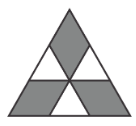2. How many zeros are there at the end of the number 201325 × 201326 × 201317 ?

3. The masses of salt and fresh water in the sea water at Protaras, Cyprus, are in the ratio 7 : 193 . How many kilograms of salt are there in 1000 kg of sea water?

4. Ann has the square sheet of paper as shown. By cutting along the lines of the square, she cuts out copies of the shape shown to the right of the square, or of the shape’s mirror image. What is the smallest possible number of cells remaining on the sheet?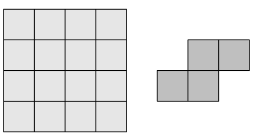5. Roo wants to tell Kanga a number with the product o f its digits equal to 24. What is the sum of the digits of the smallest number that Roo could tell Kanga?

6. A bag contains balls of the same size: two are red, three are blue, ten are white, four are green and three are black. Balls are taken from the bag w ithout looking, and not returned. What is the smallest number of balls that should be taken from the bag to be sure that two balls of the same colour have been taken?

7. Alex lights a candle every ten minutes. Each candle burns for 40 minutes and then goes out. How many candles are alight 55 minutes after Alex lit the first candle?

8. 1313.13 divided by 0.13 is equal to:

9. Mark and Liza stand on opposite sides of a circular fountain. They then start to run clockwise round the fountain. Mark's speed is 9/8 of Liza's speed. How many circuits has Liza completed when Mark catches up with her for the first time?

10. The positive integers x , y and z satisfy x × y = 14 , y × z = 10 and z × x = 35 . What is the value of x + y + z ?

11. Part B: Each correct answer is worth 4 points.

On the surface of a globe, the geography teacher drew 10 parallels and 10 meridians. Into how many areas has the surface of the globe thus been divided?

12. In the diagram, α = 55° , β = 40° and γ = 35° . What is the value of δ ?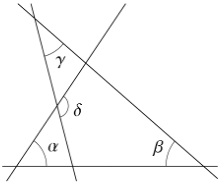13. The perimeter of a trapezoid is 5 and the lengths of its sides are integers. What are the smallest two angles of the trapezoid?

14. One of the following nets cannot be folded to form a cube. Which one?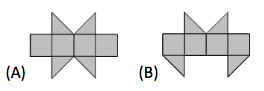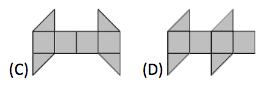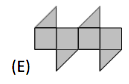15. Vasya wrote down several consecutive integers. Which of the following could not be the percentage of odd numbers among them?

16. The sides of rectangle ABCD are parallel to the coordinate axes. ABCD lies below the x -axis and to the right of the y -axis, as shown in the figure. The coordinates of t he four points A , B , C and D are all integers. For each of these points we calculate the value y -coordinate divided by the x - coordinate. Which of the four points gives the leas t value?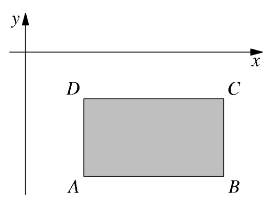17. All 4 -digit positive integers with the same four digits as in the number 2013 are written on the blackboard in an increasing order. What is the largest possible difference between two adjacent numbers on the blackboard?

18. The diagram shows a shaded quadrilateral KLMN drawn on a grid. Each cell of the grid has sides of length 2 cm . What is the area of KLMN ?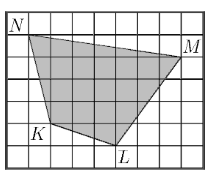19. Francois says: »On my 60th birthday I got many presents, and I hope to get as many on my 80th . Each of my children has as many children of their o wn as siblings, and none has grandchildren yet. My age is now equal to the number of all my living descendants.«
How old is Francois?

20. John has made a building of cubes standing on a 4×4 grid. The table here shows the number of cubes standing on each cell. When Joh n looks from the back, what does he see?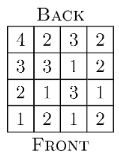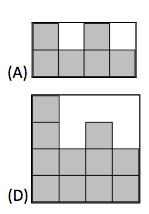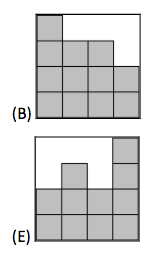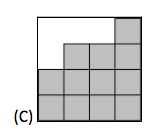21. Part C: Each correct answer is worth 5 points.

In a crypt-arithmetic puzzle, different letters rep resent different digits and the same letter always stands for the same digit. Given that the eq uality in the puzzle BDCE + BDAE = AECBE holds, what digit does the letter D represent?

22. In the 6×8 grid shown, 24 of the cells are not intersected by either diagonal. When the diagonals of a 6×10 grid are drawn, how many of the cells are not intersected by either diagonal?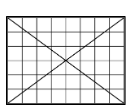23. Recall that a perfect square is a number that can be represented as a product o f two equal factors, for example 25=5 × 5=52 . A perfect cube is a number that can be represented as a product of three equal factors, for example 8=2 × 2 × 2=23 . A product of six equal factors can be written as a power , for example 26 =2 × 2 × 2 × 2 × 2 × 2 .
Let S be the number of perfect squares among the integer s from 1 to 20136 . Let Q be the number of perfect cubes among the same integers. Which of the following statements is true?

24. John chooses a 5 -digit positive integer and deletes one of its digi ts to make a 4 -digit number. The sum of this 4 -digit number and the original 5 -digit number is 52713 . What is the sum of the digits of the original 5 -digit number?

25. A gardener wants to plant twenty trees (maples and lindens) along one side of a trail in the park. The number of trees between any two maples mu st not be equal to three. Of these twenty trees, what is the greatest number of maples that the gardener can plant?

26. Four cars enter a roundabout at the same time, each one from a different direction, as shown in the diagram. Each of the car s drives less than a full round of the roundabout, and no two cars leave the roundabout in the same direction. How many different ways are there f or the cars to leave the roundabout?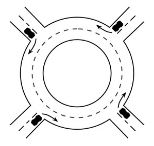27. Nick, Ryan, Simon and James from the band Dandelion sang a few songs at their first concert. Always three of them were singing, and one was playing the guitar in accompaniment. James sang the most songs of all of them – 8 . Ryan sang 5 songs, which was fewer than anyone else from the group. How many songs did Dandelion sing a t their first concert?

28. Ria bakes six raspberry pies one after the other, numbering them 1 to 6 in order, with the first being number 1 . Whilst she is doing this, her children sometimes run into the kitchen and eat the hottest pie. Which of the following could not b e the order in which the pies are eaten?

29. Each of the four vertices and six edges of a tetrah edron is marked with one of the ten numbers 1, 2, 3, 4, 5, 6, 7, 8, 9 and 11 (number 10 is omitted). Each number is used exactly once. For any two vertices of the tetrahedron, the sum of the two numbers at these vertices is equal to the number on the edge connecting these two vertices. The edge PQ is marked with the number 9 . Which number is used to mark edge RS ?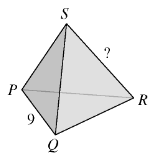30. A positive integer N is smaller than the sum of its three greatest divisors (naturally, excluding N itself). Which of the following statements is true?